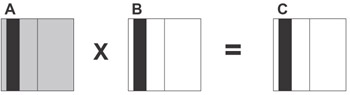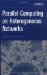# 5.2. AD HOC COMMUNICATION NETWORK

## 5.1. PROCESSORS HETEROGENEITY

### 5.1.1. Different Processor Speeds

An immediate implication from the fact that an NoC uses processors of different architectures is that the processors run at different speeds. Let us see what happens when a parallel application, which provides a good performance while running on homogeneous MPPs, runs on the cluster of heterogeneous processors.

A good parallel application for MPPs tries to evenly distribute computations over available processors. This even distribution ensures the maximal speedup on MPPs, which consist of identical processors. On the cluster of processors running at different speeds, faster processors will quickly perform their part of computations and wait for slower ones at points of synchronization. Therefore the total time of computations will be determined by the time elapsed on the slowest processor. In other words, when executing parallel applications that evenly distribute computations among available processors, the heterogeneous cluster is equivalent to a homogeneous cluster that is composed of the same number but the slowest processors.

The following simple experiment corroborates the statement. Two subnetworks of the same local network were used, each consisting of four Sun workstations. The first subnetwork included identical workstations of the same model, and was thus homogeneous. The second one included workstations of three different models. Their relative speeds, demonstrated while executing a LAPACK Cholesky factorization routine, were 1.9, 2.8, 2.8, and 7.1. As the slowest workstation (relative performance 1.9) was shared by both clusters, the total power of the heterogeneous cluster was almost twice that of the homogeneous one.

It might be expected that a parallel ScaLAPACK Cholesky solver would be executed on the more powerful cluster almost twice as fast as on the weaker one. But in reality it ran practically at the same speed (~2% speedup for a 1800 X 1800 dense matrix).

Thus a good parallel application for a NoC must distribute computa-_tions unevenly, taking into account the difference in processor speed. The faster processor is, the more computations it must perform. Ideally the volume of computation performed by a processor should be proportional to its speed.

For example, a simple parallel algorithm implementing the matrix operation C = A X B on a p-processor heterogeneous cluster, where A, B are dense square n X n matrices, can be summarized as follows:

• Each element cij in C is computed as cij = Σn-1k=0aik X bkj.

• The A, B, and C matrices are identically partitioned into p vertical slices. There is one-to-one mapping between these slices and the processors. Each processor is responsible for computing its C slice.

• Because all C elements require the same amount of arithmetic operations, each processor executes an amount of work proportional to the number of elements that are allocated to it, and hence proportional to the area of its slice. Therefore, to balance the load of the processors, the area of the slice mapped to each processor is proportional to its speed (see Figure 5.2).Figure 5.2: Matrix-matrix multiplication with matrices A, B, and C unevenly partitioned in one dimension. The area of the slice mapped to each processor is proportional to its speed. The slices mapped onto a single processor are shaded black. During execution this processor requires all of matrix A (shown shaded gray).

• To compute elements of its C slice, each processor requires all elements of the A matrix. Therefore, during the execution of the algorithm, each processor receives from p - 1 other processors all elements of their slices (shown gray in Figure 5.2).

This heterogeneous parallel algorithm cannot be implemented in HPF 1.1, since the latter provides no way to specify a heterogeneous distribution of arrays across abstract processors. But HPF 2.0 addresses the problem by extending BLOCK distribution with the ability to explicitly specify the size of each individual block (GEN_BLOCK distribution).

For example, the following HPF program implements the parallel algorithm above to multiply two dense square 1000 X 1000 matrices on a four-processor heterogeneous cluster, whose processors have the relative speeds 2, 3, 5, and 10:

`     PROGRAM HETEROGENEOUS      INTEGER, DIMENSION(4), PARAMETER:: M=(/100, 150,      250, 500/)      REAL, DIMENSION(1000,1000):: A, B, C !HPF\$  PROCESSORS p(4) !HPF\$     DISTRIBUTE (*, GEN_BLOCK(M)) ONTO p:: A, B, C !HPF\$     INDEPENDENT      DO J=1,1000 !HPF\$          INDEPENDENT           DO I=1,1000                A(I,J)=1.0                B(I,J)=2.0           END DO      END DO !HPF\$     INDEPENDENT      DO J=1,1000 !HPF\$          INDEPENDENT           DO I=1,1000                C(I,J)=0.0                DO K=1,1000                     C(I,J)=C(I,J)+A(I,K)*B(K,J)                END DO           END DO      END DO      END `

In the preceding program the “generalized” block distribution, GEN_BLOCK, is used to map contiguous segments of arrays A, B, and C of unequal sizes onto processors. The sizes of the segments are specified by values of the user-defined integer mapping array M, one value per target processor of the mapping. That is, the ith element of the mapping array specifies the size of the block to be stored on the ith processor of the target processor arrangement p. The asterisk (*) in the DISTRIBUTE directive specifies that arrays A, B, and C are not to be distributed along the first axis; thus an entire column is to be distributed as one object. So array elements A(:,1:100), B(:,1:100), and C(:,1:100) are mapped on p(1); A(:,101:250), B(:,101:250), and C(:,101:250) are mapped on p(2); A(:,251:500), B(:,251:500), and C(:,251:500) are mapped on p(3); and A(:,501:1000), B(:,501:1000); and C(:,501:1000) are mapped on p(4).

That distribution of matrices A, B, and C across processors ensures that the area of the vertical slice mapped to each processor is proportional to the speed of the processor. Note that the responsibility of the programmer is to explicitly specify the exact distribution of the arrays across processors. The specification is based on the knowledge of both the parallel algorithm and the executing heterogeneous cluster.

HPF 2.0 also allows the programmer to distribute the arrays with the REDISTRIBUTE directive based on a mapping array whose values are computed at runtime. This allows a more portable application to be written. But again, either the programmer or the user must explicitly specify the data distribution, which ensures the best performance of this particular parallel algorithm on each heterogeneous cluster.

Apparently the algorithm above can be implemented in MPI as well. The corresponding MPI program, however, will be not as simple as HPF because of the much lower level of the MPI’s programming model. Actually MPI is a programming tool of the assembler level for message-passing programming. Therefore practically all message-passing algorithms can be implemented in MPI.

Whatever programming tool is used to implement the parallel algorithm above, the efficiency of the corresponding application depends on the accuracy of estimation of the relative speed of processors of the executing heterogeneous cluster. The distribution of arrays, and hence, distribution of computations across the processors, is determined by an estimation of the relative speeds of the processors. If this estimation is not accurate enough, the load of processors will be unbalanced, resulting in poor performance.

The problem of making an accurate estimation of the relative speed of processors is not easy. For two processors of the same architecture that differ only in clock rate, it is not difficult to accurately estimate their relative speed. The relative speed will be the same for every application.

But for processors of different architectures the situation changes drastically. Everything in the processors may be different: the set of instructions, number of instruction execution units, number of registers, structure of memory hierarchy, size of each memory level, and so on and on. Therefore the processors will demonstrate different relative speeds for different applications. Moreover processors of the same architecture but different models or configurations may also demonstrate different relative speeds in different applications.

Even different applications of the same narrow class may be executed by two different processors at significantly different relative speeds. To avoid speculation, consider the following experiment. Three slightly different implementations of Cholesky factorization of a 500 X 500 matrix were used to estimate the relative speed of a SPARCstation-5 and a SPARCstation-20. Code

` for(k=0; k<500; k++) {      for(i=k, lkk=sqrt(a[k][k]); i<500; i++)           a[i][k] /= lkk;      for(j=k+1; j<500; j++)           for(i=j; i<500; i++)                a[i][j] -= a[i][k]*a[j][k]; }`

estimated their relative speed as 10_:_9, meanwhile code

` for(k=0; k<500; k++) {      for(i=k, lkk=sqrt(a[k][k]); i<500; i++)           a[i][k] /= lkk;      for(i=k+1; i<500; i++)           for(j=i; j<500; j++)                a[i][j] -= a[k][j]*a[k][i]; }`

as 10:14. Routine dptof2 from the LAPACK package, solving the same problem, estimated their relative speed as 10:_10.

### 5.1.2. Heterogeneity of Machine Arithmetic

Since processors of an NoC may do floating-point arithmetic differently, there are special challenges associated with writing numerical software on NoCs. In particular, two basic issues potentially affect the behavior of a numerical parallel application running on a heterogeneous NoC.

First, different processors do not guarantee the same storage representation and the same results for operations on floating point numbers. Second, if a floating-point number is communicated among processors, the communication layer does not guarantee the exact transmittal of the floating-point value. Normally transferring a floating point number in a heterogeneous environment includes two conversions of its binary representation: the representation of the number on the sender site is first converted into a machine independent representation, which is then converted into the representation for floating-point numbers on the receiver site. The two successive conversions may change the original value; that is, the value received by the receiver may differ from the value sent by the sender.

To see better the potential problems, consider the iterative solution of a system of linear equations where the stopping criterion depends on the value of some function, f, of the relative machine precision, e. A common definition of the relative machine precision, or unit roundoff, is the smallest positive floating-point value, e, such that fl(1 + e) > 1, where fl(x) is the floating-point representation of x. The test for convergence might well include a test of the form

` if  goto converged;`

In a heterogeneous setting the value of f may be different on different processors and er and xr may depend on data of different accuracies. Thus one or more processes may converge in a fewer number of iterations. Indeed, the stopping criterion used by the most accurate processor may never be satisfied if it depends on data computed less accurately by other processors. If the code contains communication between processors within an iteration, it may not complete if one processor converges before the others. In a heterogeneous environment, the only way to guarantee termination is to have one processor make the convergence decision and broadcast that decision.

Another problem is that overflow and underflow exceptions may occur during floating-point representation conversions, resulting in a failure of the communication.Parallel Computing on Heterogeneous Networks (Wiley Series on Parallel and Distributed Computing)
ISBN: B000W7ZQBO
EAN: N/A
Year: 2005
Pages: 95